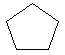Email us to get an instant 20% discount on highly effective K-12 Math & English kwizNET Programs!

Online Quiz (WorksheetABCD)

Questions Per Quiz = 2 4 6 8 10

Grade 5 - Mathematics7.11 Regular Polygons

 A polygon in which all the sides are equal and all the angles are equal is called a regular polygon. Directions: Answer the following. Also draw at least five examples of regular polygon.
 Q 1: The figure shows regular triangle which is also calledright angle triangleisosceles triangleequilateral triangle Q 2: The figure shown is a regularoctagonpentagonhexagon Question 3: This question is available to subscribers only! Question 4: This question is available to subscribers only!